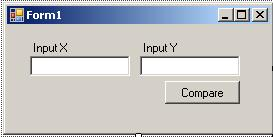Kodyaz Development Resources Development resources, articles, tutorials, code samples and tools and downloads for ASP.Net, SQL Server, R Script, Windows, Windows Phone, AWS, SAP HANA and ABAP, like SAP UI5, Screen Personas, etc.
 Home Articles News IT Jobs Tools Sample Chapters Trainers Blogs Forums Photos Files

.NET Framework, ASP.NET

ASP.NET, Windows Forms, Controls, .NET Framework and Visual Studio Articles

ASP.NET Forums

Visual Studio Forums

.NET Development Blog

Certification Exams Blog

# Conditional Statements

Conditional statements are part of program flow statements that provides the software developer build a complex logical structure within codes. Conditional statements evaluates a condition and produces a true or a false boolean value in order to control the flow of code.

The first conditional statement for every developer to remember first will be the "If ... Then ... Else". Another conditional statement is the "Switch" statement in C# or its equivalent "SELECT CASE" in VB.Net

Let's make a few samples with the conditional statements in order to review the usage of these statements.

## If ... Then ... Else

If...Then...Else statement executes a group of code according to the boolean value of an expression in the condition statement. The condition statement is evaluated for a boolean value which is True or False, and if condition generates a True value then the first block of code is run. If there is an ELSE part of the If...Then...Else block then the second block of code is executed for a False generated value of condition.

If you look at the syntax of the If...Then...Else conditional statement, it will be more clear to understand the flow of the code

If if_condition Then
statements
[ ElseIf elseif_condition Then
elseif_statements ]
[ Else
else_statements ]
End If

For example, assume that we design a form where users can enter input values for two integer variables and compare their values.In the Click event of the button, we can run a similar code block for evaluating the input values

``` Dim x, y As Integer Try x = CInt(txtInputX.Text) y = CInt(txtInputY.Text) Catch ex As Exception MessageBox.Show("enter integer values for input variables") Exit Sub End Try If x < y Then MessageBox.Show(String.Format("Input x ({0}) is smaller then input y ({1})", x, y)) ElseIf x = y Then MessageBox.Show(String.Format("Input x ({0}) is equal to input y ({1})", x, y)) Else MessageBox.Show(String.Format("Input x ({0}) is bigger then input y ({1})", x, y)) End If ```

You can also run a single line mode of the If...Then...Else statement. (Note that the If statement is in single line but may be displayed in multi lines on this article because of screen width issues)

``` Dim message As String If x < y Then message = "Input x ({0}) is smaller then input y ({1})" Else If x = y Then message = "Input x ({0}) is equal to input y ({1})" Else message = "Input x ({0}) is bigger then input y ({1})" MessageBox.Show(String.Format(message, x, y)) ```

## Select...Case

Select...Case statement is very useful if you have a list of possible values. The Select...Case statement evaluates an expression and then compares the results of the expression with possible result lists. Then it runs codes depending to which possible result matches the condition.

Select Case testexpression
Case expressionlist
statements
[ Case Else
elsestatements ]
End Select

Let's re-write our sample code using the Select...Case statement

``` Select Case x.CompareTo(y) Case -1 MessageBox.Show(String.Format("Input x ({0}) is smaller then input y ({1})", x, y)) Case 0 MessageBox.Show(String.Format("Input x ({0}) is equal to input y ({1})", x, y)) Case 1 MessageBox.Show(String.Format("Input x ({0}) is bigger then input y ({1})", x, y)) Case Else MessageBox.Show(String.Format("Input x ({0}) and input y ({1}) can not be compared!", x, y)) End Select ```

A good thing about the Select...Case is that you can compare a range of values or a list of values in one Case expressionlist

``` Select Case x Case 1 MessageBox.Show(String.Format("Input x ({0}) is equal to 1", x)) Case 2, 3, 4, 5 MessageBox.Show(String.Format("Input x ({0}) is one of the numbers 2,3,4 and 5", x)) Case 6 To 10 MessageBox.Show(String.Format("Input x ({0}) is between 6 and 10 including 6 and 10", x)) Case Else MessageBox.Show(String.Format("Please try a number between 1 to 10", x)) End Select ```

You can apply the range of list values for string values also. For string comparison alphabetical comparison is done to set whether a string value is between the upper and lower limits of the range.